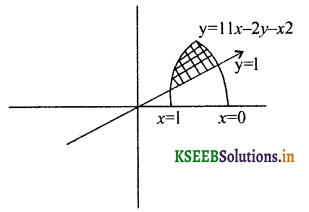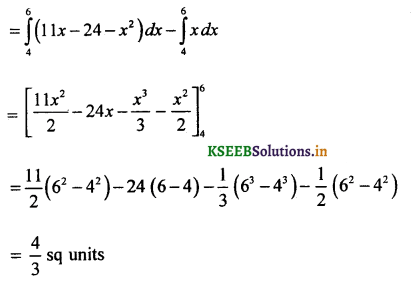# 2nd PUC Basic Maths Question Bank Chapter 21 Definite Integral and its Applications to Areas

Students can Download Basic Maths Question Bank Chapter 21 Definite Integral and its Applications to Areas Questions and Answers, Notes Pdf, 2nd PUC Basic Maths Question Bank with Answers helps you to revise the complete Karnataka State Board Syllabus and to clear all their doubts, score well in final exams.

## Karnataka 2nd PUC Basic Maths Question Bank Chapter 21 Definite Integral and its Applications to Areas

### 2nd PUC Basic Maths Definite Integral and its Applications to Areas One Mark Questions and Answers

Question 1.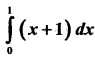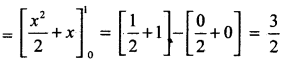Question 2.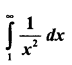Question 3.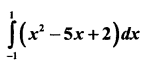Question 4.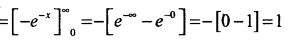Question 5.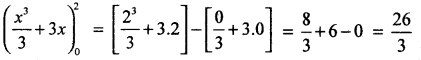Question 6.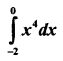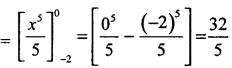Question 7.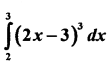Question 8.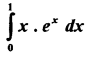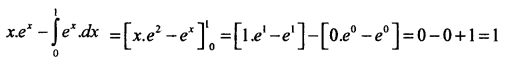Question 9.Question 10.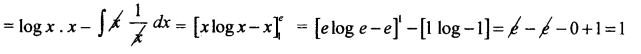Question 11.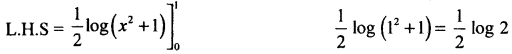Question 12.Question 13.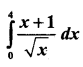Question 14.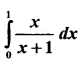Question 15.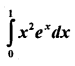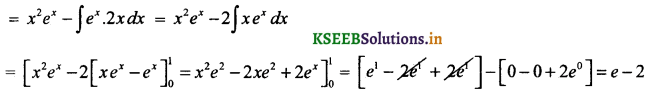Question 16.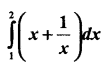Question 17.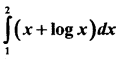Question 18.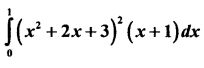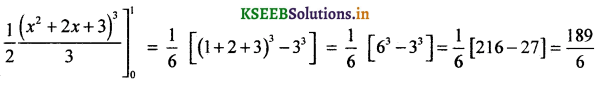Question 19.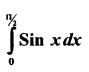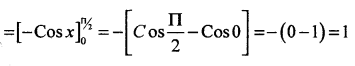Question 20.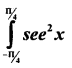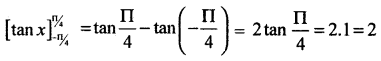Question 21.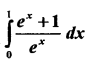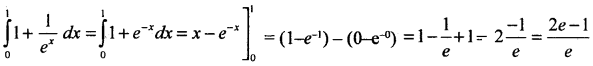Question 22.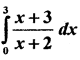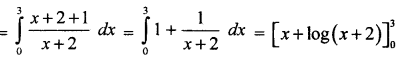= 3 + log(3 + 2) – 0 +1og(0 + 2) = 3 + log 5 – log 2 = 3 + log $$\frac { 5 }{ 2 }$$Question 23.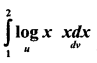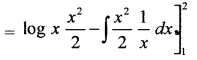Question 24.Question 25.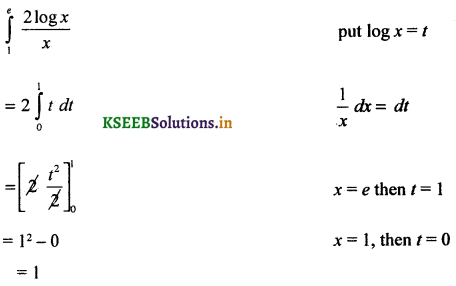Question 26.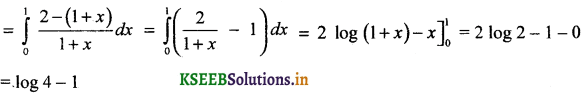Question 27.
If marginal cost = x2 – x – 2. Find the total cost obtained from an output of 40 unitsQuestion 28.
If the marginal revenue is given by f1(R) = 15 – $$\frac{x}{15}$$ find the Total revenue obtoined from an output of 30 units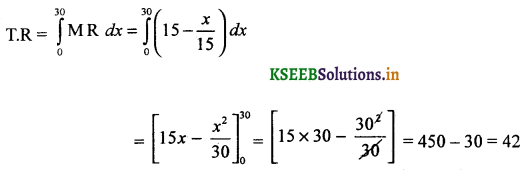Question 29.
If the marginal cost of a firm is f1(x) 10 + 6x – 6x2 where xis the output find the total cost among that the fixed cost is ₹ 125.
TC = ∫MC dx
∫10 + 6x – 6x2 dx
= 10x + $$\frac{6 x^{2}}{2}-\frac{6 x^{3}}{3}$$ + C given C = 125 = fixed cost
= 10x + 3x2 – 2x3 + 125

Question 30.
If the M R is f1(x) = 20 Find the total revenue & average revenue obtained from an output of 30 units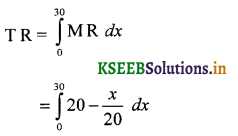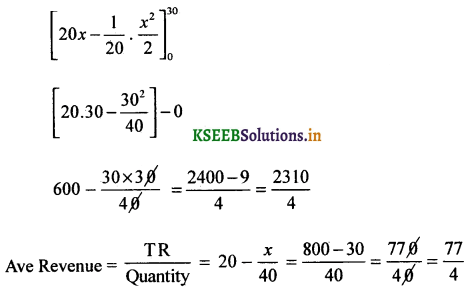Question 31.
Compute the total cost for the marginal cost function f1(x) = 3x2 – 6x + 12 assuming that the fixed cost is ₹500 Also find Average cost
T C ∫MC dx
Where fixed cost C 500 Rs.
= ∫(326x + 12)dx = 3$$\frac{x^{3}}{3}$$ – 6x$$\frac{x^{2}}{2}$$ + 12x + C = x3 – 3x2 + 12x + 500
Ave cost = $$\frac{\mathrm{TC}}{x}$$
$$\frac{x^{3}-3 x^{2}+12 x+500}{x}$$
x2 – 3x + 12 + $$\frac{500}{x}$$

Question 32.
If the M R = 16 – x2 find the maximun total Revenue Also find the total & the average reveues also write the demand function
TR ∫MR dx
= ∫(16 – x2)dz
l6x – $$\frac{x^{3}}{3}$$ + C when output = 0,
TR = 16x – $$\frac{x^{3}}{3}$$ Total revenue = 0 ∴ C = 0
Average revenue = $$\frac{\mathrm{TR}}{x}=\frac{16 x-\frac{x^{3}}{3}}{x}=16-\frac{x^{2}}{3}$$
Demand function = 16 – $$\frac{x^{3}}{3}$$

Question 33.
The marginal cost function is f1(x) = 3x2 + 2x + 1 wherex is the level of output find the total cost, ave cost, total variable cost, average variable cost given that fixed cost 70
TC ∫MCdx
= ∫(3x2 + 2x + 1)
= 3 $$\frac{x^{3}}{3}$$ + 2$$\frac{x^{2}}{2}$$ + x + C given fixed cost C = 70
= x3 + x2 + x + 70
Total variable cost = 2x3 + x2 + x
Average cost = $$\frac{\text { Total cost }}{x}$$
= $$\frac{x^{3}+x^{3}+x+70}{x}$$ = x3 + x + 1 + $$\frac{70}{x}$$
Average variable cost = 2x3 + x + 1Question 34.
Find the area bounded by the curve y = x2, x – axis and the ordinates are x = 0, x = 1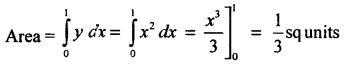Question 35.
Find the area bounded by the curve y2 = 8x, x – axis & the lines x = 0, x = 2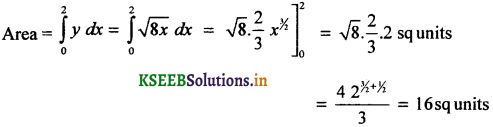Question 36.
Find the area bounded by the curve x2 = 8y, y – axis and abscissas y = 3. y = 6 ∵ x = $$\sqrt{8 y}=\sqrt{8} \cdot \sqrt{y}$$### 2nd PUC Basic Maths Definite Integral and its Applications to Areas Five Marks Questions and Answers

Question 1.
Find the area between the curves y2 = 4x & x2 = 4y
Given y2 = 4x but y = $$\frac{x^{2}}{4}$$
$$\left(\frac{x^{2}}{4}\right)^{2}$$ = 4x
$$\frac{x^{4}}{16}$$ = 4x ⇒ x4 – 64x = 0 ⇒ x(x3 – 43) = 0
⇒ x = 0 or x = 4
Required Area A = |A1 – A2|Question 2.
Find the area bounded by the curve y2 = 5x and the line y = x
y2 = 5x and y = x
⇒ x2 = 5x
⇒ x2 = 5x
⇒ x2 – 5x = 0
⇒ x(x – 5) = 0 ⇒ x = 0 or x = 5 are the limits
Required Area A = |A1 – A2|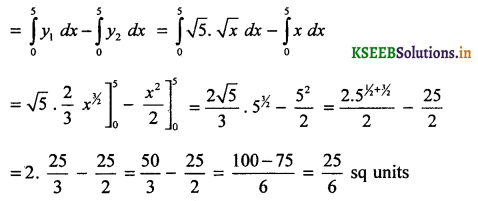Question 3.
Find the area between the curves x2 = 5y & y = 2x.
Given x2 = 5y & y = 2x
∴ x2 = 5 (2x)
x2 = 10x ⇒ x(x – 10) = 0 ⇒ x = 0 or x = 10
RequiredArea = A = |A1 – A2|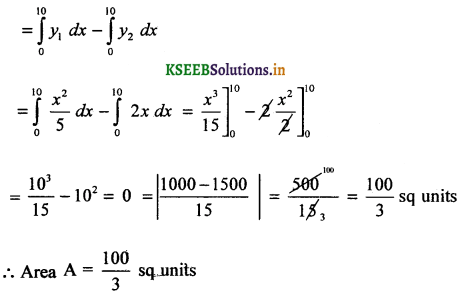Question 4.
Find the area bounded by the parabolas x2 = y & y2 = 8x
Given y2 = 8 & y = x2
∴ (x2)2 = 8x
x4 – 8x = 0
⇒ x(x3 – 23) =0 ⇒ x = 0 or x = 2
RequiredArea A = |A1 – A2|Question 5.
Find the ares exciosed between the parabola y2 = x and the Iine x + y = 2
Given y = 2 – x & y2 = x
⇒ (2 – x)2 = x
4 + x2 – 4x – x = 0
x2 – 5x + 4 = 0
x = 4 or 1 (both are +v)
If x = 4, y = 2 – x = 2 – 4 = 2
If x = 1, y = 2 – x = 2 – 1 = 1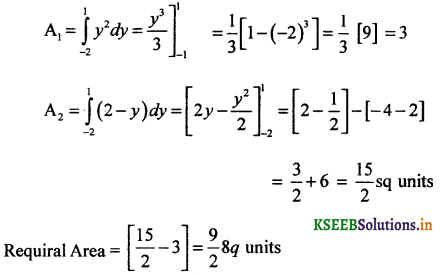Question 6.
Find the area between the parabolas y2 = 4x & the line y = x
Given y2 = 4x & y = x
⇒ x2 = 4x
⇒ x(x – 4) = 0 ⇒ x = 0 or x = 4
Required area A = A1 – A2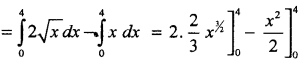Question 7.
Find the area between the cures y = 8 – x2 & y = x2
Given y = 8 – x2 & y = x2
x2 = 8 – x2
2x2 = 8 ⇒ x2 = 4 ⇒ x = ±2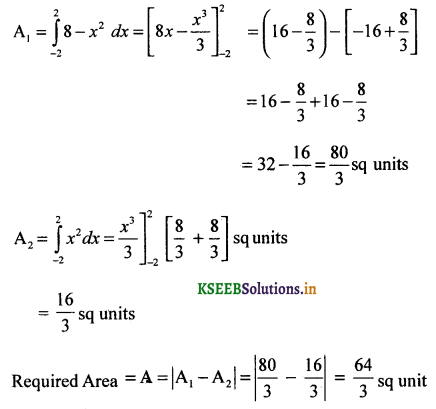Question 8.
Find the area bounded by the parabola y2 = 4ax & its latus rectum
Required Area = 2 Area OAB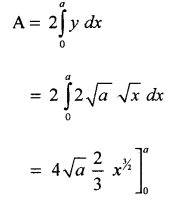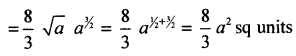Question 9.
Find the arca exclosed between the parabola x2 = 4y and the line x = 4y – 2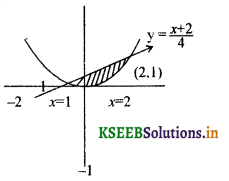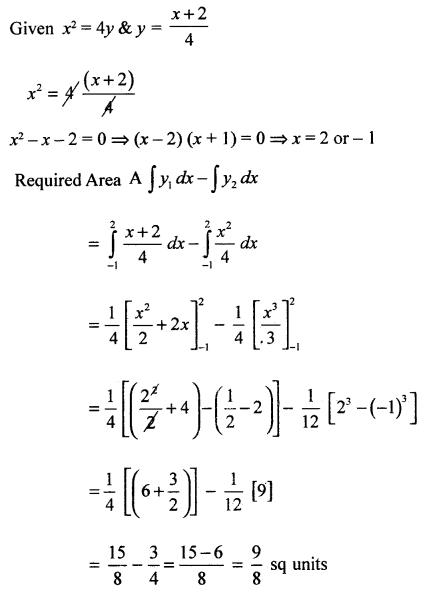Question 10.
Find the area exciosed between y = 11x – 24 – x2 & the line y = x